Next: Singular Value Decomposition Up: Algorithms for Principal Component Previous: Algorithms for Principal Component

### Principal subspace and components

Assume that we have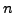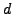-dimensional data vectors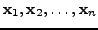, which form the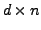data matrix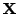=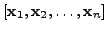. The matrixis decomposed into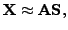(1)

where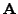is a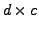matrix,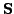is a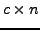matrix and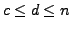. Principal subspace methods [6,4] findandsuch that the reconstruction error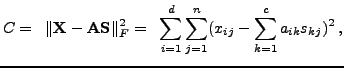(2)

is minimized. Theredenotes the Frobenius norm, and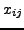,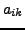, and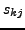elements of the matrices,, and, respectively. Typically the row-wise mean is removed fromas a preprocessing step.

Without any further constraints, there exist infinitely many ways to perform such a decomposition. However, the subspace spanned by the column vectors of the matrix, called the principal subspace, is unique. In PCA, these vectors are mutually orthogonal and have unit length. Further, for each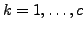, the first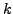vectors form the-dimensional principal subspace. This makes the solution practically unique, see [4,2,5] for details.

There are many ways to determine the principal subspace and components [6,4,2]. We will discuss three common methods that can be adapted for the case of missing values.Next: Singular Value Decomposition Up: Algorithms for Principal Component Previous: Algorithms for Principal Component
Tapani Raiko 2007-09-11